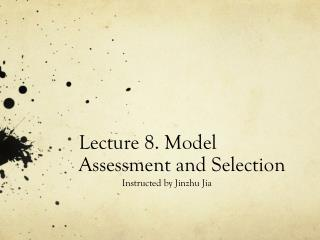Download PresentationLecture 8. Model Assessment and Selection

Loading in 2 Seconds...

# Lecture 8. Model Assessment and Selection - PowerPoint PPT Presentation

Lecture 8. Model Assessment and Selection. Instructed by Jinzhu Jia. Outline. Introduction – Generalization Error Bias-Variance Decomposition Optimism of Training Error Rate AIC, BIC, MDL Cross Validation Bootstrap. What we will learn?.I am the owner, or an agent authorized to act on behalf of the owner, of the copyrighted work described.
Download Presentation## Lecture 8. Model Assessment and Selection

An Image/Link below is provided (as is) to download presentation

Download Policy: Content on the Website is provided to you AS IS for your information and personal use and may not be sold / licensed / shared on other websites without getting consent from its author.While downloading, if for some reason you are not able to download a presentation, the publisher may have deleted the file from their server.

- - - - - - - - - - - - - - - - - - - - - - - - - - E N D - - - - - - - - - - - - - - - - - - - - - - - - - -
Presentation Transcript
1. Lecture 8. Model Assessment and Selection Instructed by Jinzhu Jia

2. Outline • Introduction – Generalization Error • Bias-Variance Decomposition • Optimism of Training Error Rate • AIC, BIC, MDL • Cross Validation • Bootstrap

3. What we will learn? • Assessment of generalization performance: prediction capability on independent test data • Use this assessment to select models

4. Loss Function • Y: target variable • X: predictors, inputs • prediction model that is estimated from a training set • : Loss function.

5. Test Error • Test error, also referred to as generalization error • Here the training set is fixed, and test error refers to the error for this specific training set. • Expected prediction error: • Training error:

6. Behavior of Errors Red: conditional test error Blue: train error

7. Categorical data • -2loglikelihood is referred to deviance

8. General response densities Example: The loss above is just a quadratic loss.

9. Ideal Situation for Performance Assessment • Enough data • Train – for fitting • Validation – for estimate prediction error used for Model selection • Test– for assessment of the generalization error of the final chosen model

10. What if insufficient data? • Approximate generalization error via AIC, BIC, CV or Bootstrap

11. The Bias-Variance Decomposition • Typically, the more complex we make the model , the lower the bias, but the higher the variance.

12. Bias-Variance Decomposition • For the k-nearest-neighbor regression fit, • For linear fit, In-sample error:

13. Bias-variance Decomposition

14. Example: Bias-variance Tradeoff • 80 obs, 20 predictors ~ U[0,1]^20

15. Example: Bias-variance Tradeoff Expected prediction error Squared bias variance

16. Optimism of the Training Error Rate • Given a training set • Generalization error is • Note: training set is fixed, while is a new test data point • Expected error:

17. Optimism of the Training Error rate • Training error will be less than test error • Hence, training error will be an overly optimistic estimate of the generalization error.

18. Optimism of the Training Error Rate • In-sample Error: • Generally speaking, op > 0 • Average optimism:

19. Estimate of In-sample Prediction Error For linear fit with d predictors: AIC =

20. The Bayesian approach and BIC Gaussian model Laplace approximation

21. Cross Validation

22. Cross Validation Prediction Error Ten-fold CV

23. GCV • For linear fit:

24. The wrong way to do CV

25. The Right Way

26. Bootstrap

27. Bootstrap

28. Bootstrap

29. Conditional or Expected Test Error

30. Homework • Due May 16 • ESLII_print 5, pp216. Exercise 7.3, 7.9, 7.10, Reproduce Figure 7.10